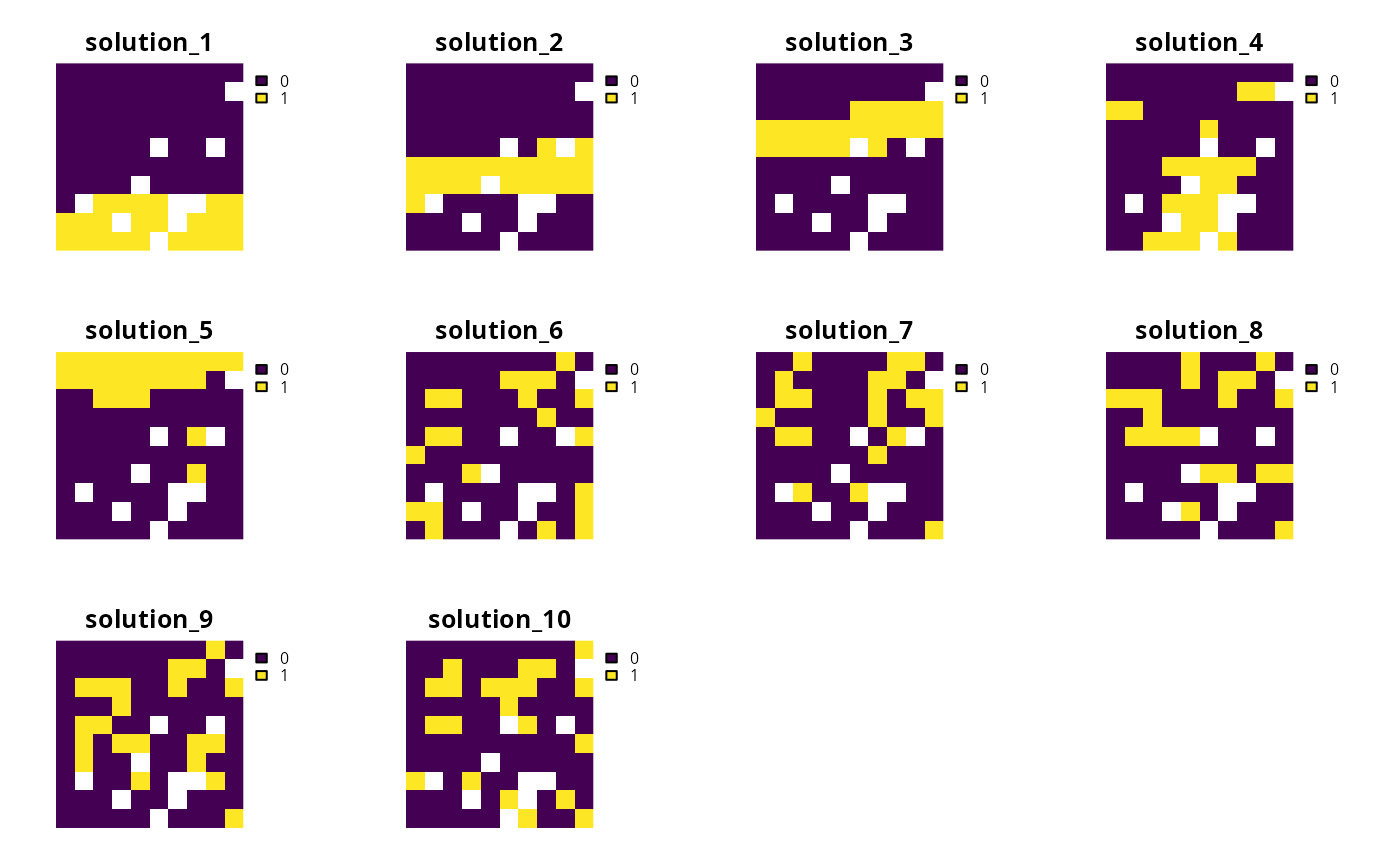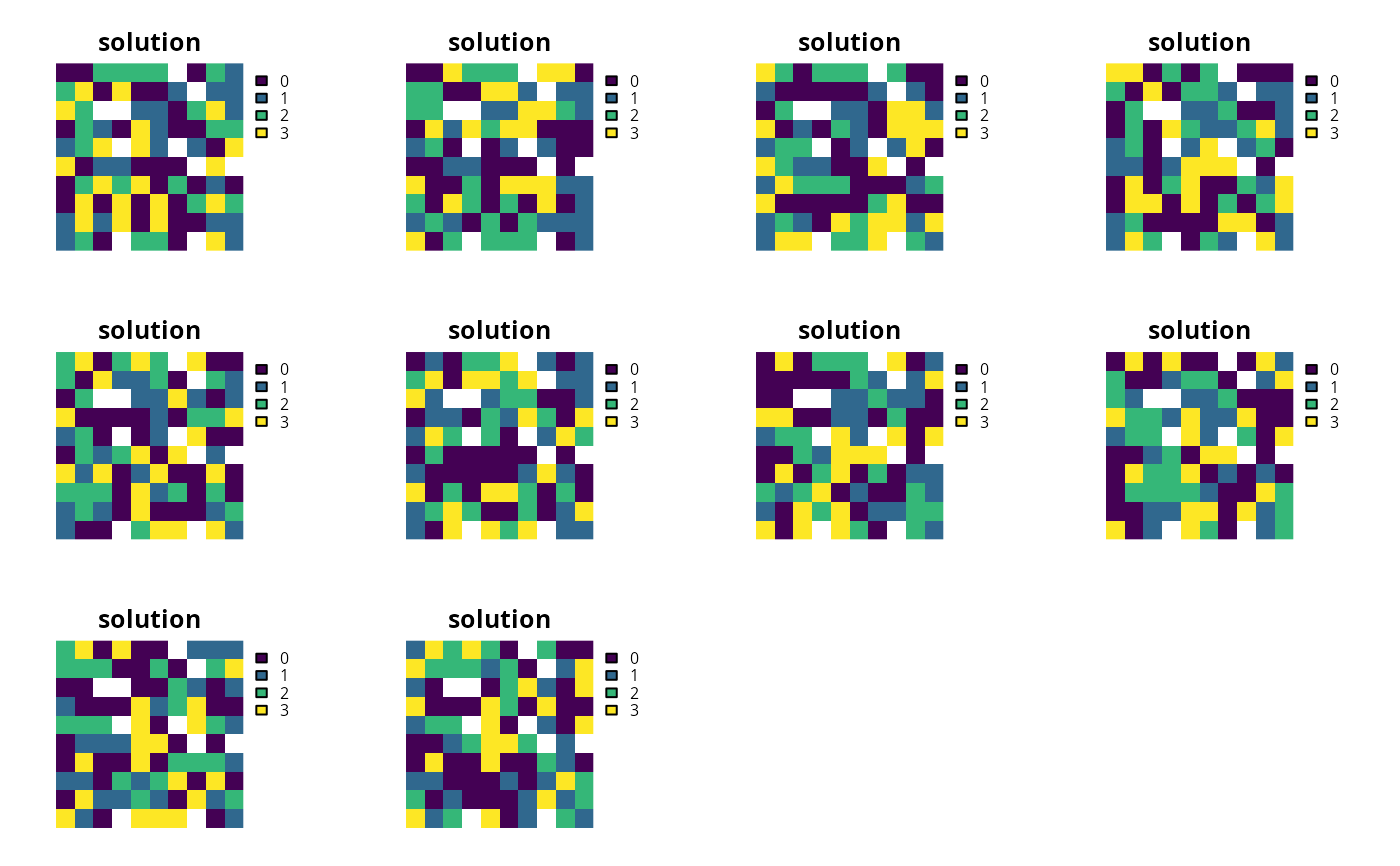Generate a portfolio of solutions for a conservation planning problem() using Bender's cuts (discussed in Rodrigues et al. 2000). This is recommended as a replacement for add_gap_portfolio() when the Gurobi software is not available.

add_cuts_portfolio(x, number_solutions = 10L)

## Arguments

x

problem() (i.e., ConservationProblem) object.

number_solutions

integer number of attempts to generate different solutions. Defaults to 10.

## Value

Object (i.e., ConservationProblem) with the portfolio added to it.

## Details

This strategy for generating a portfolio of solutions involves solving the problem multiple times and adding additional constraints to forbid previously obtained solutions. In general, this strategy is most useful when problems take a long time to solve and benefit from having multiple threads allocated for solving an individual problem.

See portfolios for an overview of all functions for adding a portfolio.

Other portfolios: add_extra_portfolio(), add_gap_portfolio(), add_shuffle_portfolio(), add_top_portfolio()

## Examples

# set seed for reproducibility
set.seed(500)

data(sim_pu_raster, sim_features, sim_pu_zones_stack, sim_features_zones)

# create minimal problem with cuts portfolio
p1 <- problem(sim_pu_raster, sim_features) %>%
add_default_solver(gap = 0.2, verbose = FALSE)

# \dontrun{
# solve problem and generate 10 solutions within 20% of optimality
s1 <- solve(p1)

# plot solutions in portfolio
plot(stack(s1), axes = FALSE, box = FALSE)# }
# build multi-zone conservation problem with cuts portfolio
p2 <- problem(sim_pu_zones_stack, sim_features_zones) %>%
add_relative_targets(matrix(runif(15, 0.1, 0.2), nrow = 5,
ncol = 3)) %>%
add_default_solver(gap = 0.2, verbose = FALSE)

# \dontrun{
# solve the problem
s2 <- solve(p2)

# print solution
str(s2, max.level = 1)
#> List of 10
#>  $solution_1 :Formal class 'RasterStack' [package "raster"] with 11 slots #>$ solution_2 :Formal class 'RasterStack' [package "raster"] with 11 slots
#>  $solution_3 :Formal class 'RasterStack' [package "raster"] with 11 slots #>$ solution_4 :Formal class 'RasterStack' [package "raster"] with 11 slots
#>  $solution_5 :Formal class 'RasterStack' [package "raster"] with 11 slots #>$ solution_6 :Formal class 'RasterStack' [package "raster"] with 11 slots
#>  $solution_7 :Formal class 'RasterStack' [package "raster"] with 11 slots #>$ solution_8 :Formal class 'RasterStack' [package "raster"] with 11 slots
#>  $solution_9 :Formal class 'RasterStack' [package "raster"] with 11 slots #>$ solution_10:Formal class 'RasterStack' [package "raster"] with 11 slots
#>  - attr(*, "objective")= Named num [1:10] 11601 11815 11790 11820 11618 ...
#>   ..- attr(*, "names")= chr [1:10] "solution_1" "solution_2" "solution_3" "solution_4" ...
#>  - attr(*, "status")= Named chr [1:10] "OPTIMAL" "OPTIMAL" "OPTIMAL" "OPTIMAL" ...
#>   ..- attr(*, "names")= chr [1:10] "solution_1" "solution_2" "solution_3" "solution_4" ...
#>  - attr(*, "runtime")= Named num [1:10] 0.01 0.008 0.048 0.009 0.009 ...
#>   ..- attr(*, "names")= chr [1:10] "solution_1" "solution_2" "solution_3" "solution_4" ...

# plot solutions in portfolio
plot(stack(lapply(s2, category_layer)), main = "solution", axes = FALSE,
box = FALSE)# }